Miscellaneous

Chapter 13 Class 11 Statistics
Serial order wise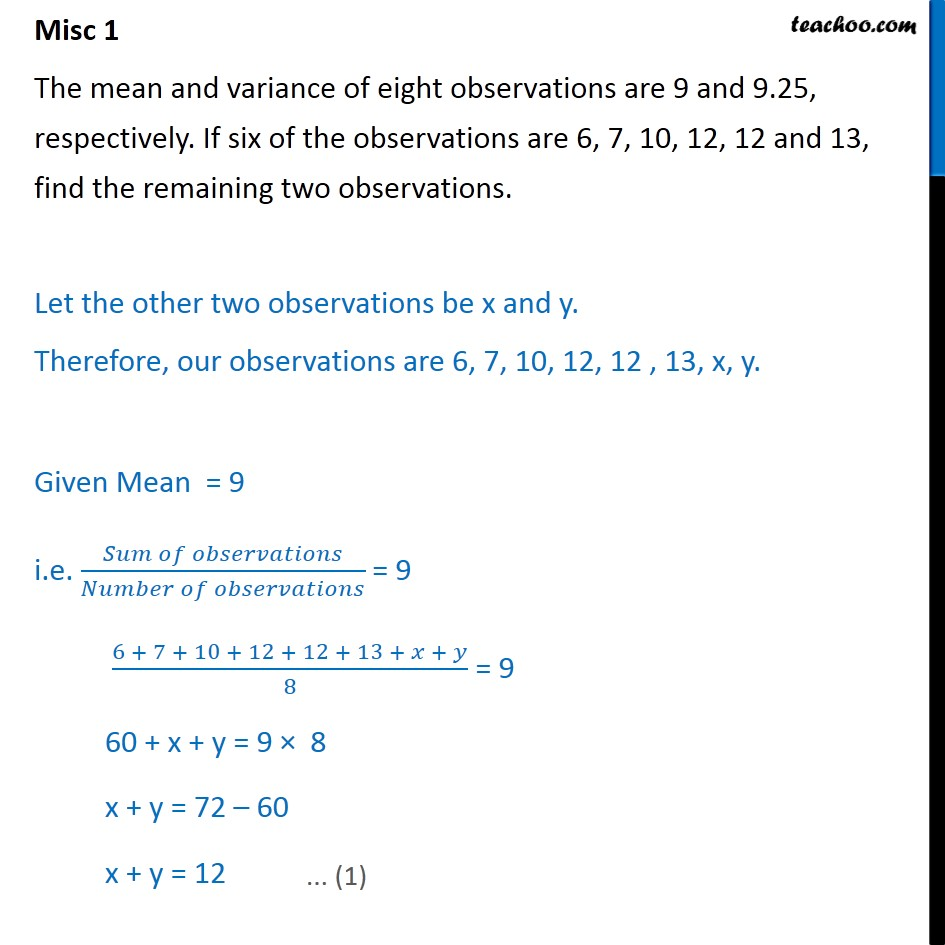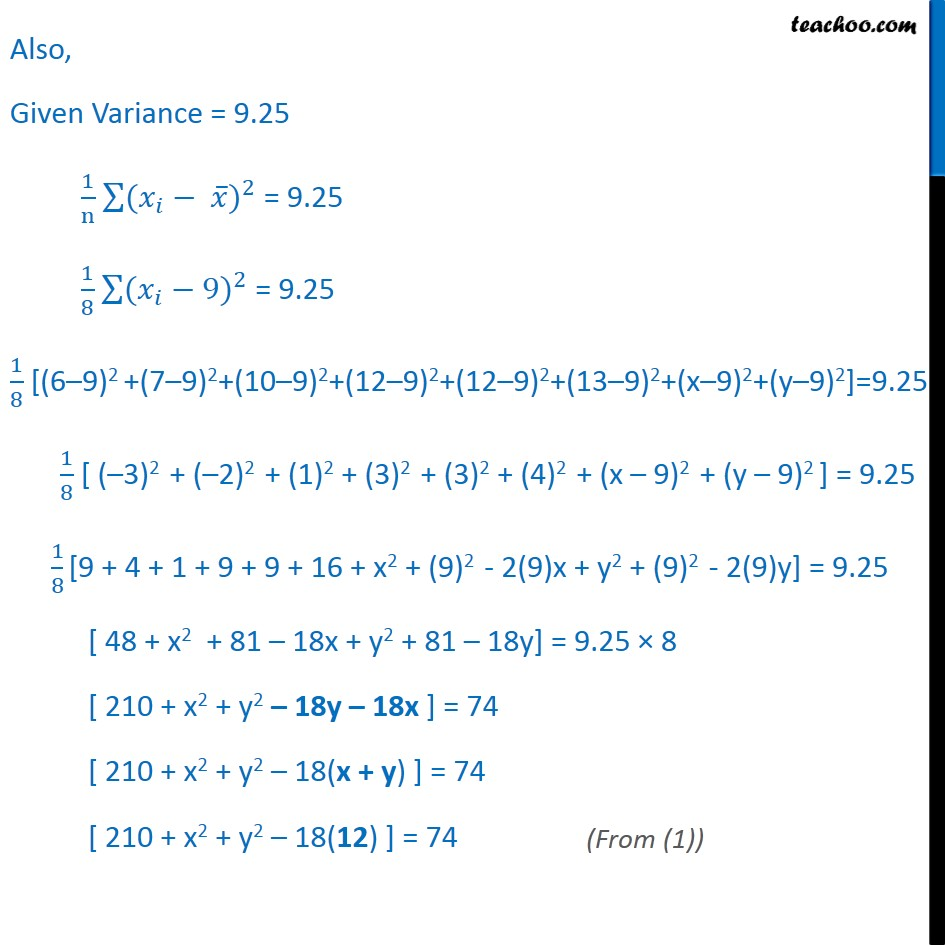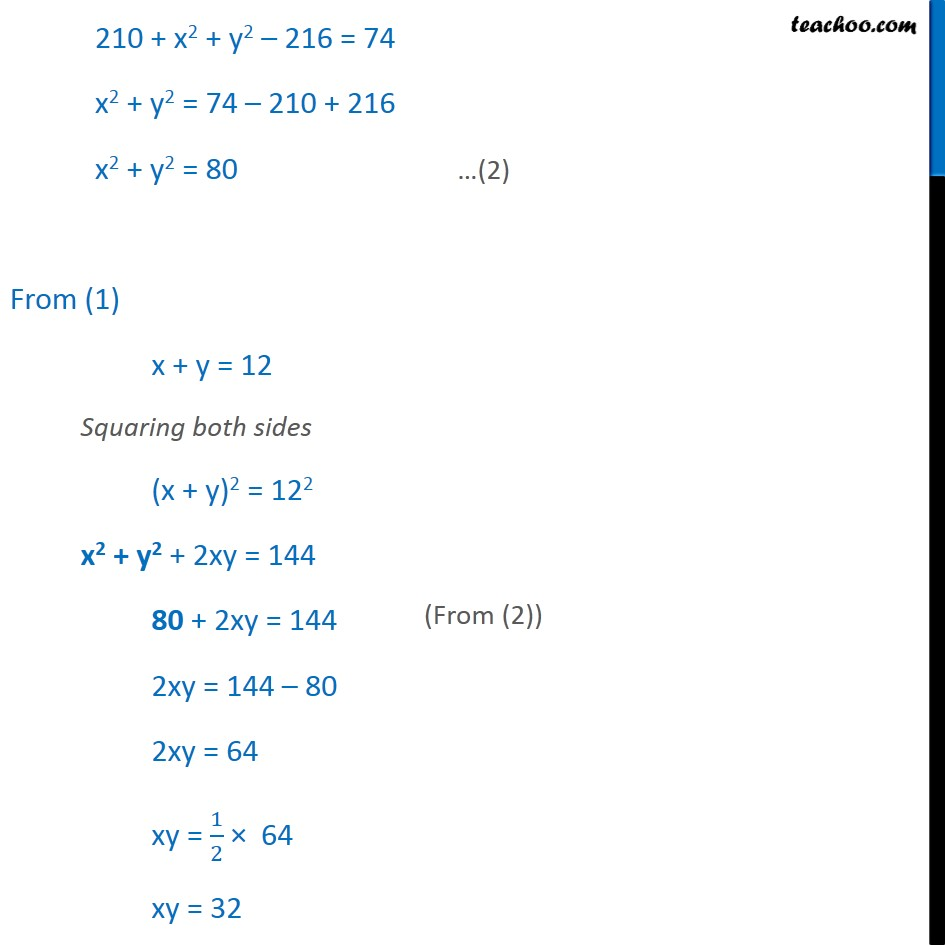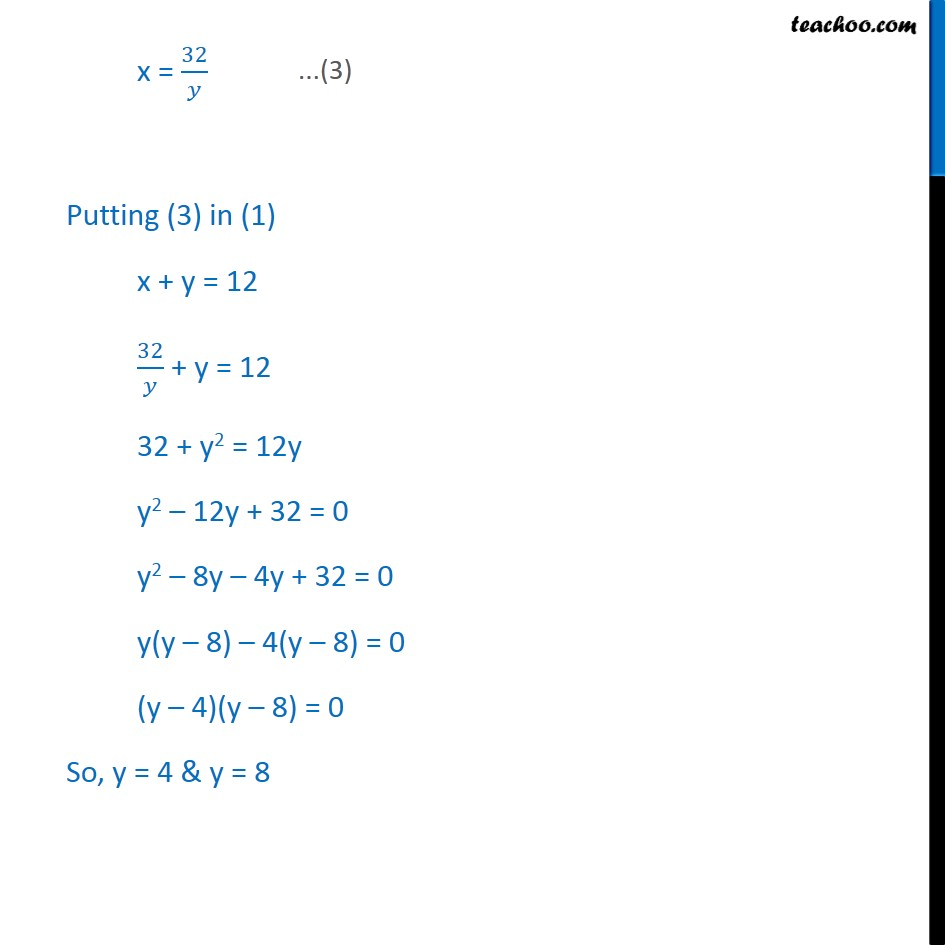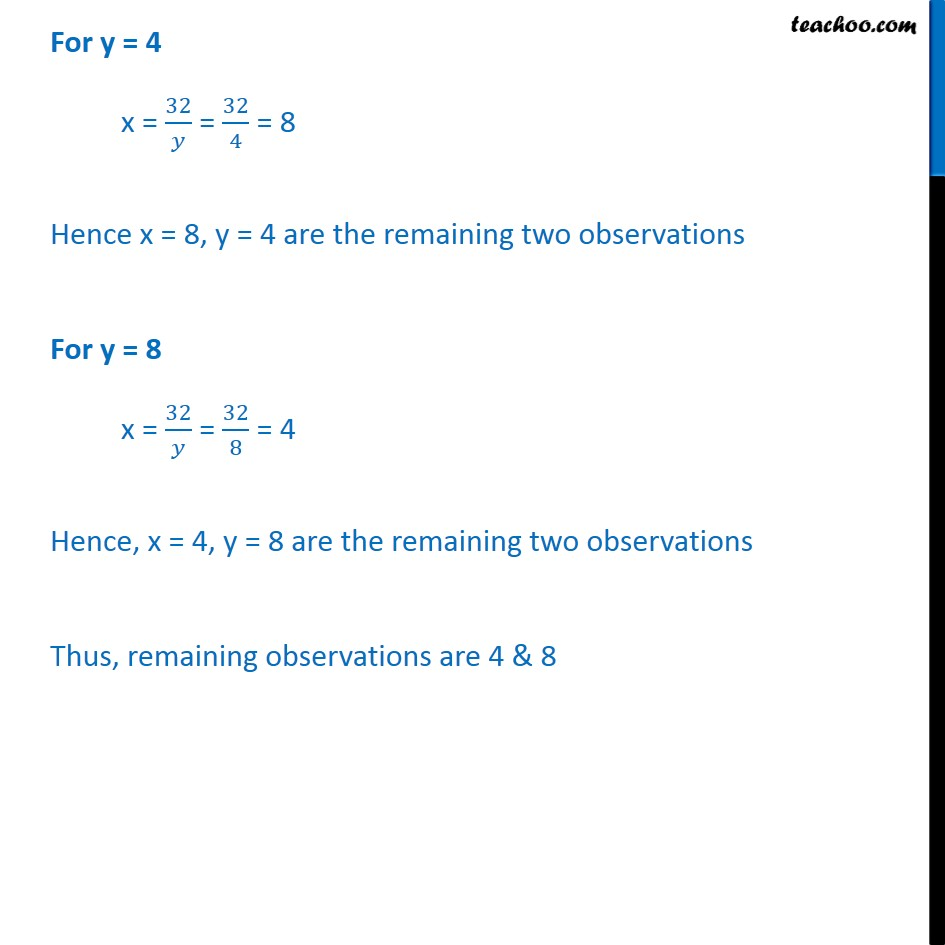Learn in your speed, with individual attention - Teachoo Maths 1-on-1 Class

### Transcript

Misc 1 The mean and variance of eight observations are 9 and 9.25, respectively. If six of the observations are 6, 7, 10, 12, 12 and 13, find the remaining two observations. Let the other two observations be x and y. Therefore, our observations are 6, 7, 10, 12, 12 , 13, x, y. Given Mean = 9 i.e. 𝑆𝑢𝑚 𝑜𝑓 𝑜𝑏𝑠𝑒𝑟𝑣𝑎𝑡𝑖𝑜𝑛𝑠﷮𝑁𝑢𝑚𝑏𝑒𝑟 𝑜𝑓 𝑜𝑏𝑠𝑒𝑟𝑣𝑎𝑡𝑖𝑜𝑛𝑠﷯ = 9 6 + 7 + 10 + 12 + 12 + 13 + 𝑥 + 𝑦﷮8﷯ = 9 60 + x + y = 9 × 8 x + y = 72 – 60 x + y = 12 Also, Given Variance = 9.25 1﷮n﷯ ﷮﷮( 𝑥﷮𝑖﷯﷯− 𝑥﷯)﷮2﷯ = 9.25 1﷮8﷯ ﷮﷮( 𝑥﷮𝑖﷯﷯−9)﷮2﷯ = 9.25 1﷮8﷯ [(6–9)2 +(7–9)2+(10–9)2+(12–9)2+(12–9)2+(13–9)2+(x–9)2+(y–9)2]=9.25 1﷮8﷯ [ (–3)2 + (–2)2 + (1)2 + (3)2 + (3)2 + (4)2 + (x – 9)2 + (y – 9)2 ] = 9.25 1﷮8﷯ [9 + 4 + 1 + 9 + 9 + 16 + x2 + (9)2 - 2(9)x + y2 + (9)2 - 2(9)y] = 9.25 [ 48 + x2 + 81 – 18x + y2 + 81 – 18y] = 9.25 × 8 [ 210 + x2 + y2 – 18y – 18x ] = 74 [ 210 + x2 + y2 – 18(x + y) ] = 74 [ 210 + x2 + y2 – 18(12) ] = 74 210 + x2 + y2 – 216 = 74 x2 + y2 = 74 – 210 + 216 x2 + y2 = 80 From (1) x + y = 12 Squaring both sides (x + y)2 = 122 x2 + y2 + 2xy = 144 80 + 2xy = 144 2xy = 144 – 80 2xy = 64 xy = 1﷮2﷯ × 64 xy = 32 x = 32﷮𝑦﷯ Putting (3) in (1) x + y = 12 32﷮𝑦﷯ + y = 12 32 + y2 = 12y y2 – 12y + 32 = 0 y2 – 8y – 4y + 32 = 0 y(y – 8) – 4(y – 8) = 0 (y – 4)(y – 8) = 0 So, y = 4 & y = 8 For y = 4 x = 32﷮𝑦﷯ = 32﷮4﷯ = 8 Hence x = 8, y = 4 are the remaining two observations For y = 8 x = 32﷮𝑦﷯ = 32﷮8﷯ = 4 Hence, x = 4, y = 8 are the remaining two observations Thus, remaining observations are 4 & 8